Math Calculators, Lessons and Formulas

It is time to solve your math problem

mathportal.org
• Geometry
• Rhombus and Parallelogram

# Rhombus and Parallelogram

ans:
syntax error
C
DEL
ANS
±
(
)
÷
×
7
8
9
4
5
6
+
1
2
3
=
0
.
auto next question
calculator
•  Question 1: 1 pts Is the following expression true or false? $$\beta = 116^{\circ}$$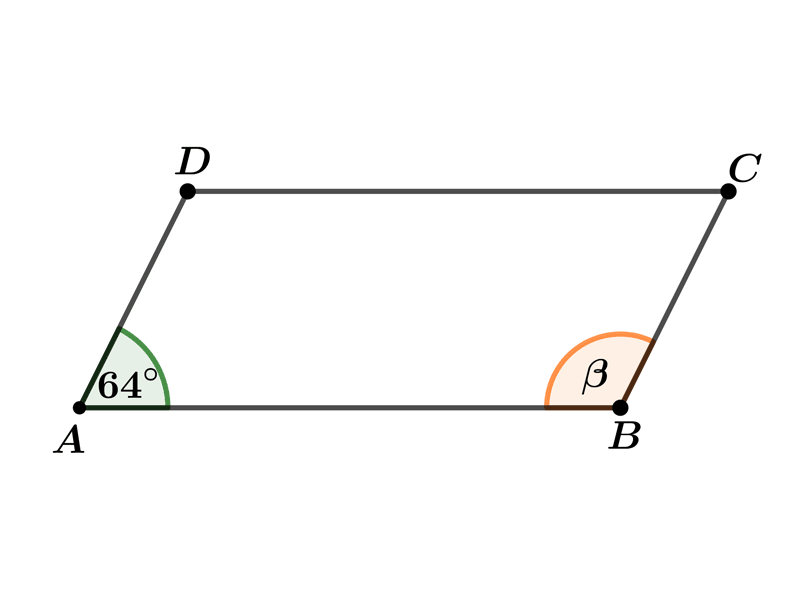•  Question 2: 1 pts Is the followin expression true or falsr? $$x=90^{\circ}$$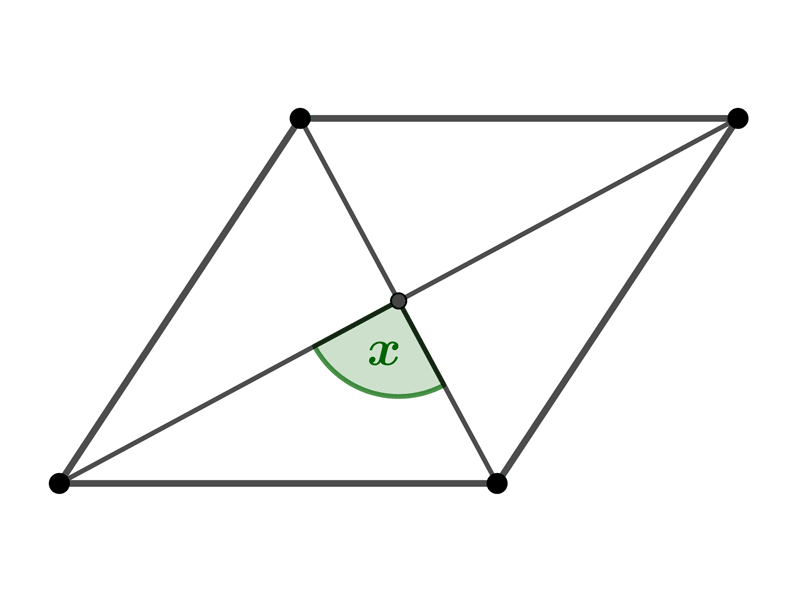•  Question 3: 1 pts Find the length of the side of the rhombus shown on the picture.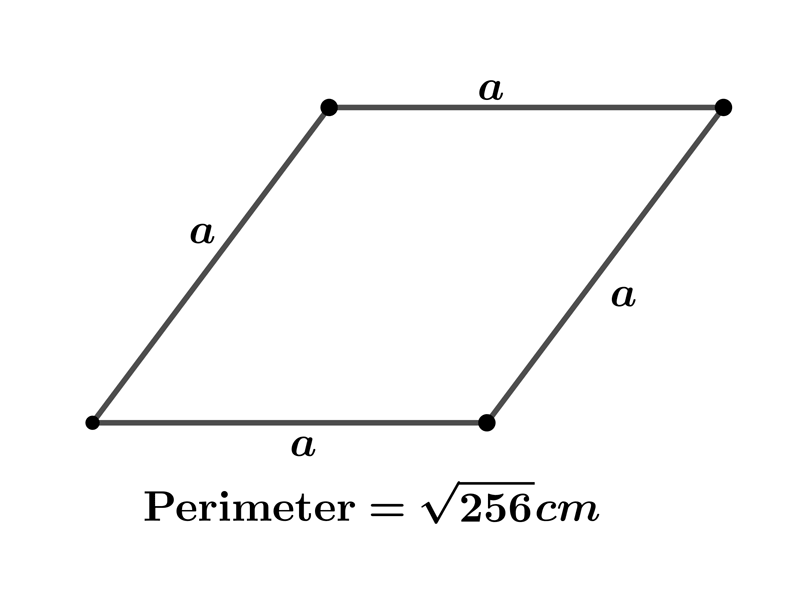$a=8cm$ $a=11cm$ $a=6cm$ $a=4cm$
•  Question 4: 1 pts Is the following expression true or false? $$\sphericalangle CAD \cong \sphericalangle BAC$$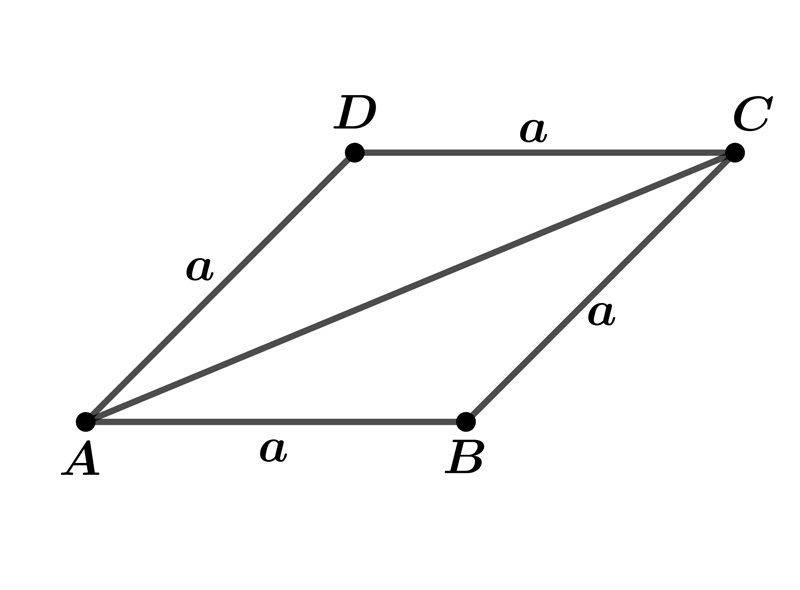•  Question 5: 1 pts The length for one side of the rhombus shown on the picture is $14cm.$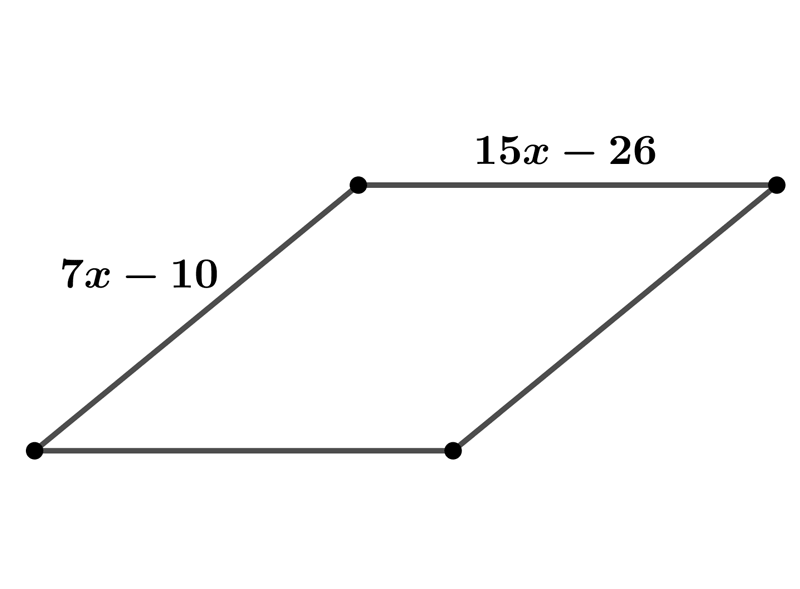•  Question 6: 2 pts Find the length of the side of a rhombus whose diagonals are $6cm$ and $2\sqrt{7}cm$?
 $a=7cm$ $a=5cm$ $a=4cm$ $a=6cm$
•  Question 7: 2 pts Find the area of a rhombus whose one diagonal is $16 cm$ and the length of one side of the rhombus is $17cm$?
 $A=240cm^{2}$ $A=240\sqrt{5}cm^{2}$ $A=840cm^{2}$ $A=2\sqrt{14}cm^{2}$
•  Question 8: 2 pts Find the perimeter of the rhombus shown on the picture.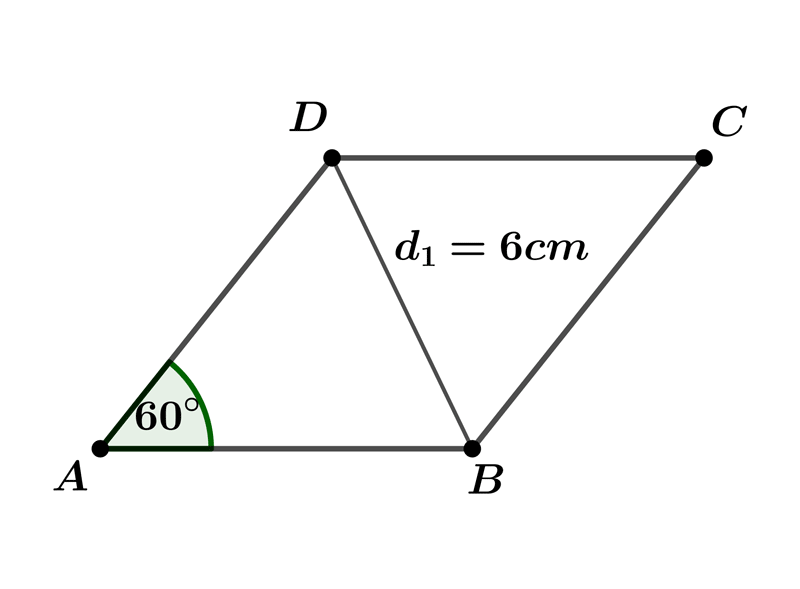$P=$
•  Question 9: 2 pts $ABCD$ is a rhombus with side length 4 cm. One diagonals has a length of 6.4cm. Find the height of that rhombus.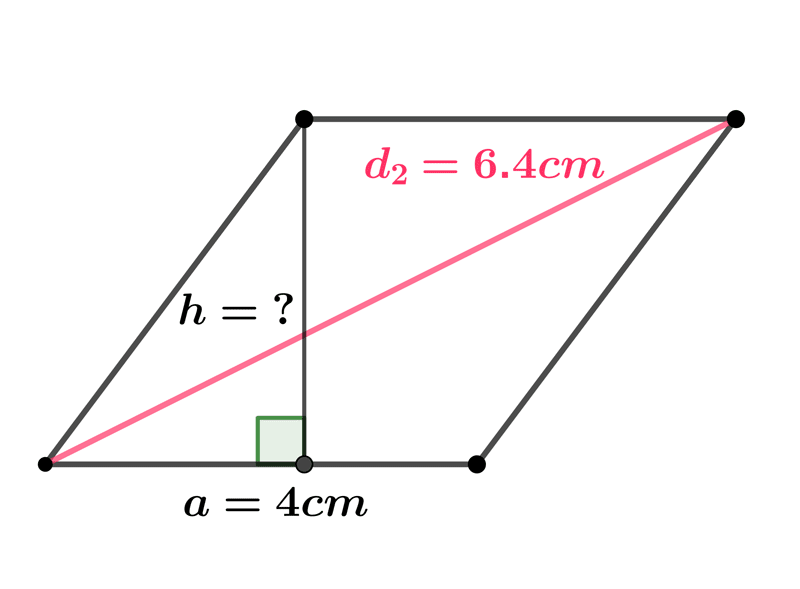Height$=$
•  Question 10: 3 pts It is given a rhombus of side length $a = 10 cm$, and the length one of its diagonal is $16 cm.$ Calculate the radius $R$of inscribed circle shown on the picture.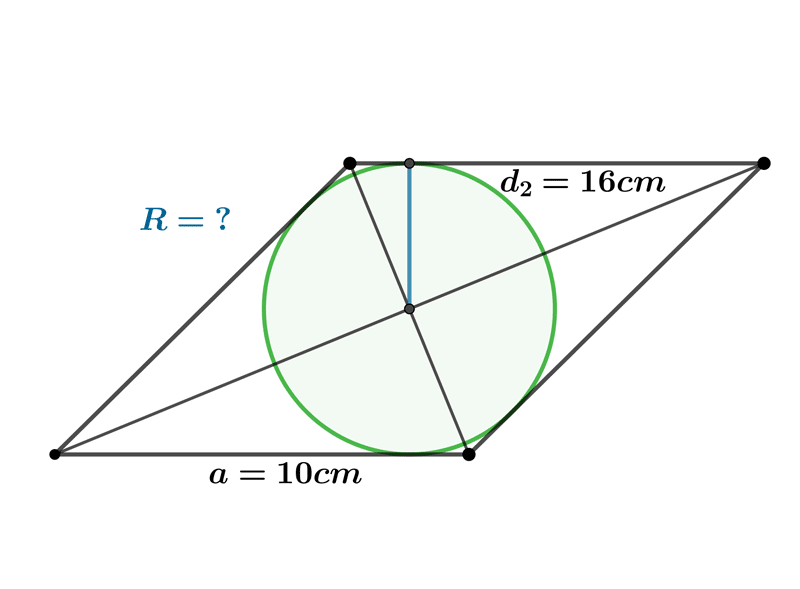$R=$
•  Question 11: 3 pts The diagonals of a rhombus are in the ratio $3:4.$ If the perimeter is $60cm$ find the area of the rhombus.
 $A=108cm^{2}$ $A=216cm^{2}$ $A=324cm^{2}$ $A=444cm^{2}$
•  Question 12: 3 pts Find the missing value $x$.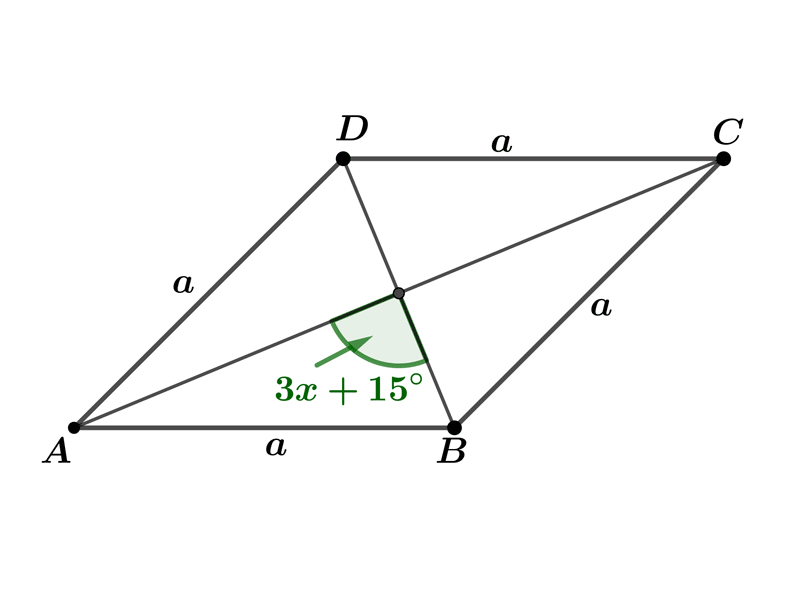$x=45^{\circ}$ $x=25^{\circ}$ $x=95^{\circ}$ $x=72^{\circ}$